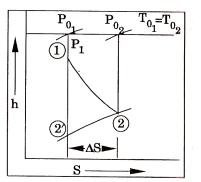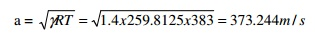Home | | Gas Dynamics and Jet Propulsion | Important Questions and Answers: Isentropic Flows

# Important Questions and Answers: Isentropic Flows

Mechanical - Gas Dynamics and Jet Propulsion - Basic Concepts and Isentropic Flows

GAS DYNAMICS AND JET PROPULSION

1.What is the basic difference between compressible and incompressible fluid flow?

Compressible

1.       Fluid velocities are appreciable compared  with  the  velocity  of sound

2.       Density is not constant

3.       Compressibility factor is greater than one.

Incompressible

1.       Fluidvelocitiesaresmall compared  with  the  velocity  of sound

2.       Density is constant

3.       Compressibility factor is one.

2. Write the steady flow energy equation for an adiabatic flow of air.

In an adiabatic flow q = 0.  Therefore energy equation becomes.3. Define the mach number in terms of bulk modulus of elasticity.

Mach number is a non-dimensional number and is used for the analysis of compressible fluid flows.4. Explain the meaning of stagnation state with example.

The state of a fluid attained by isentropically decelerating it to zero velocity at zero elevation is referred as stagnation state.

(e.g.) Fluid in a reservoir (or) in a settling chamber.

5. Distinguish between static and stagnation pressures.

In stagnation pressure state, the velocity of the flowing fluid is zero whereas in the static pressure state, the fluid velocity is not equal to zero.

6. Differentiate between the static and stagnation temperatures.

The actual temperature of the fluid in a particular state is known as “static temperature” whereas the temperature of the fluid when the fluid velocity is zero at zero elevation is known as “stagnation temperature”.7. What is the use of mach number?

Mach number is defined as the ratio between the local fluid velocity to the velocity of  sound.

i.e. Mach number

M =   Local fluid velocity /  Velocity of sound = c/ a

It is used for the analysis of compressible fluid flow problems. Critical mach number is a dimensionless number at which the fluid velocity is equal to its sound velocity. Therefore,Crocco number is a non – dimensional fluid velocity which is defined as the ratio of

fluid velocity to its maximum fluid velocity.8. Write down the relationship between stagnation and static temperature interms of the flow, mach number for the case of isentropic flow.T0= stagnation temperature

T= Static temperature

M= Mach number.

9.Give the expression of  p/P0  for an isentropic flow through a duct.

The expression of10. Name the four reference velocities that are used in expressing the fluid velocities in

non-dimensional form?11.            What are the different regions of compressible flow.The adiabatic energy equation for a perfect gas is derived in terms of fluid velocity © and sound velocity (a). This is then plotted graphically on the c- a co-ordinates, a steady flow ellipse is obtained.The various regions of flow are:

i.Incompressible region (M 0)

(ii)              Subsonic region     (M < 1)

(iii)             Transonic region   (0.8 – 1.2)

(iv)             Supersonic region  (M > 1 and M < 5)

(v)              Hypersonic region (M ≥ 5)

12.  Define  M*  and  give  the  relation  between  M

and M*.

It is a non-dimensional mach number and is defined by the ratio between the local fluid velocity to its critical velocity of sound / fluid.13.  If  an  aeroplane  goes  to  higher  altitudes  maintaining  the  same  speed,  the  Mach

number will remain constant.  Say true or false.

False.

W.K.T. M = c/a

At higher altitude, the sound velocity ‘a’ will decrease and hence M will increase. Therefore, M is not constant.

14.  Show  h  –  S  diagram  for  the  flow  through  a  nozzle.   Show  how  the  stagnation

properties get affected.

1 – 2’ = Isentropic expansion

1 – 2 = Adiabatic expansionIt is assumed that, the exit pressure is same for both  cases.   But  stagnation  pressure  at  the  exit of the adiabatic  process   (P02  ) will be  less than  isentropic pressure (P02  ' ) . This   is due   to friction   and irresversibilities.  But stagnation temperature remains constant.

15. A plane travels at a speed of 2400 KM/h in an atmosphere of 5°C, find the mach angle.16.Define mach angle and mach wedge.Mach angle is formed, when an object is moving with supersonic speed. The wave propagation and changes are smooth. When an object is moving with hypersonic speed the changes are abrupt is shown in Fig. Hence for a supersonic flow over two – dimensional object “mach wedge” is used instead of “mach cone”.

17. How will you illustrate the role of mach number as a measure of compressibility?

If the flow is assumed to be incompressible, the value of pressure co-efficient (or) compressibility factor obtained by Bernoulli equation is unity.By substituting different values of M, we can get different values of compressibility factor and is given in the table.

In the above table, when M increases, the compressibility factor also increases from the initial value 1. Thus the role of mach number is a measure of compressibility.

18. What is meant by isentropic flow with variable area?

A steady one dimensional isentropic flow in a variable area passages is called “variable area flow”. The heat transfer is negligible and there are no other irreversibilities due to fluid friction, etc.

19.            Define zone of action and zone of silence with neat sketch.

20.Find the sonic velocity in oxygen when it is at 110° C, γ=1.4 and molecular weight 32.Study Material, Lecturing Notes, Assignment, Reference, Wiki description explanation, brief detail
Mechanical : Gas Dynamics and Jet Propulsion : Basic Concepts and Isentropic Flows : Important Questions and Answers: Isentropic Flows |